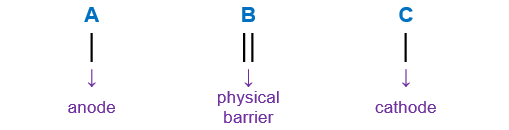# Problem: A voltaic cell prepared using aluminum and nickel has the following cell notation.Al(s) | Al3+(aq) || Ni2+(aq) | Ni(s)Which of the following reactions occurs at the anode?A) Al(s) → Al3+(aq) + 3e–B) Al3+(aq) + 3e → Al(s)C) Ni(s) → Ni2+(aq) + 2e–D) Ni2+(aq) + 2e– → Ni(s)E) none of the above

###### FREE Expert Solution

We’re being asked to write  reactions occurs at the anode for the given cell notation below:

Al(s) | Al3+(aq) || Ni2+(aq) | Ni(s)

When writing a cell notation, we use the following format – “as easy as ABCRecall the mnemonics LEO GER.

95% (228 ratings)###### Problem Details

A voltaic cell prepared using aluminum and nickel has the following cell notation.

Al(s) | Al3+(aq) || Ni2+(aq) | Ni(s)

Which of the following reactions occurs at the anode?

A) Al(s) → Al3+(aq) + 3e

B) Al3+(aq) + 3e → Al(s)

C) Ni(s) → Ni2+(aq) + 2e

D) Ni2+(aq) + 2e → Ni(s)

E) none of the above